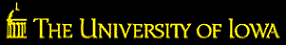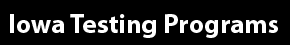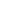Our sites: Primary Website | Login to Online Tools | Login to Bar Coding | Login to eITP# IEOC Algebra II Content Coverage

The Iowa End-of-Course assessments are designed to assess student knowledge and skills in a variety of subject areas. The test consists of 30 multiple-choice items and is designed to be administered during a single class period. The test items, which are aligned to the Common Core State Standards, reflect educator input and a rigorous quality-control process to ensure appropriateness, content accuracy, and clarity.

A summary of the content covered within the Algebra II test is presented below.

## Number and Quantity The Real Number System

Topics may include using properties of rational exponents and using properties of rational and irrational numbers.

## The Complex Number System

Topics may include performing arithmetic operations with complex numbers, representing complex numbers and their operations on the complex plane, and using complex numbers in polynomial identities and equations.

## Algebra Seeing Structure in Expressions

Topics may include interpreting the structure of expressions and writing expressions in equivalent forms to solve problems.

## Arithmetic with Polynomials and Rational Expressions

Topics may include performing arithmetic operations on polynomials, understanding the relationship between zeros and factors of polynomials, using polynomial identities to solve problems, and rewriting rational expressions.

## Reasoning with Equations and Inequalities

Topics may include solving linear equations and inequalities, quadratic equations, rational equations, and radical equations in one variable; solving systems of equations; and graphing linear equations and inequalities, and polynomial, rational, absolute value, exponential, and logarithmic functions.

## Functions Interpreting Functions

Topics may include using function notation and analyzing linear, quadratic, square root, cube root, piecewise-defined, polynomial, rational, exponential, and logarithmic functions.

## Building Functions

Topics may include creating functions describing relationships between quantities, using arithmetic operations on functions, composing functions, translating between explicit and recursive forms of sequences, understanding the effects of function transformations, finding inverse functions, and understanding and using the inverse relationship between exponents and logarithms to solve problems.

## Linear, Quadratic, and Exponential Models

Topics may include constructing and comparing linear, quadratic, and exponential functions; interpreting different representations of linear and exponential functions, including the parameters of the functions in terms of the context represented; and evaluating logarithms as solutions of exponential functions.